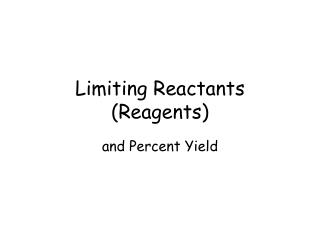# Limiting Reactants (Reagents) - PowerPoint PPT PresentationDownload PresentationLimiting Reactants (Reagents)

Limiting Reactants (Reagents)Download Presentation## Limiting Reactants (Reagents)

- - - - - - - - - - - - - - - - - - - - - - - - - - - E N D - - - - - - - - - - - - - - - - - - - - - - - - - - -
##### Presentation Transcript

1. Limiting Reactants (Reagents) and Percent Yield

2. Calculations need to be based on the limiting reactant. • Example 1: Suppose a box contains 87 bolts, 110 washers and 99 nails. How many sets of 1 bolt, 2 washers and 1 nail can you use to create? What is the limiting factor? 55 sets; washers limit the amount

3. Calculations need to be based on the limiting reactant. • Example 2: What is the maximum mass of sulfur dioxide that can be produced by the reaction of 95.6 g carbon disulfide with 100. g oxygen? CS2 + O2 CO2 + SO2 Start by balancing the equation…

4. Calculations need to be based on the limiting reactant. • Example 2: What is the maximum mass of sulfur dioxide that can be produced by the reaction of 95.6 g carbon disulfide with 100. g oxygen? CS2 + 3O2 CO2 + 2SO2 Now solve the problem…

5. Calculations need to be based on the limiting reactant. • Example 2: What is the maximum mass of sulfur dioxide that can be produced by the reaction of 95.6 g carbon disulfide with 100. g oxygen? CS2 + 3O2 CO2 + 2SO2 Which reactant is LIMITING?

6. Calculations need to be based on the limiting reactant. • Example 2: What is the mass of sulfur dioxide that can be produced by the reaction of 95.6 g carbon disulfide with 100. g oxygen? CS2 + 3O2 CO2 + 2SO2 O2 limits the amount of SO2 that can be produced. CS2 is in excess.

7. Calculations need to be based on the limiting reactant. • Example 2: What is the mass of sulfur dioxide that can be produced by the reaction of 95.6 g carbon disulfide with 100. g oxygen? CS2 + 3O2 CO2 + 2SO2 134 g of SO2 can be produced in this reaction.

8. Calculations need to be based on the limiting reactant. • Example 3: What mass of CO2 could be formed by the reaction of 8.0 g CH4 with 48 g O2? CH4 + O2 CO2 + H2O Start by balancing the equation…

9. Calculations need to be based on the limiting reactant. • Example 3: What mass of CO2 could be formed by the reaction of 8.0 g CH4 with 48 g O2? CH4 + 2O2 CO2 + 2H2O Now solve the problem…

10. Calculations need to be based on the limiting reactant. • Example 3: What mass of CO2 could be formed by the reaction of 8.0 g CH4 with 48 g O2? CH4 + 2O2 CO2 + 2H2O Which reactant is LIMITING?

11. Calculations need to be based on the limiting reactant. • Example 3: What mass of CO2 could be formed by the reaction of 8.0 g CH4 with 48 g O2? CH4 + 2O2 CO2 + 2H2O CH4 limits the amount of CO2 that can be produced. O2 is in excess.

12. Many chemical reactions do not go to completion (reactants are not completely converted to products). Percent Yield: indicates what percentage of a desired product is obtained.

13. So far, the masses we have calculated from chemical equations were based on the assumption that each reaction occurred 100%. • The THEORETICAL YIELD is the yield calculated from the balance equation. • The ACTUAL YIELD is the amount “actually” obtained in an experiment.

14. Look back at Example 2. We found that 134 g of SO2 could be formed from the reactants. • In an experiment, you formed 130 g of SO2. What is your percent yield?

15. Example: A 10.0 g sample of ethanol, C2H5OH, was boiled with excess acetic acid, CH3COOH, to produce 14.8 g of ethyl acetate, CH3COOC2H5. What percent yield of ethyl acetate is this? CH3COOH + C2H5OH  CH3COOC2H5 + H2O

16. Example: A 10.0 g sample of ethanol, C2H5OH, was boiled with excess acetic acid, CH3COOH, to produce 14.8 g of ethyl acetate, CH3COOC2H5. What percent yield of ethyl acetate is this?